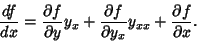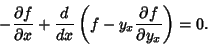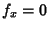## Beltrami Identity

An identity in Calculus of Variations discovered in 1868 by Beltrami. The Euler-Lagrange Differential Equation is(1)

Now, examine the Derivative of(2)

Solving for theterm gives(3)

Now, multiplying (1) bygives(4)

Substituting (3) into (4) then gives(5)(6)

This form is especially useful if, since in that case(7)

which immediately gives(8)

whereis a constant of integration.

The Beltrami identity greatly simplifies the solution for the minimal Area Surface of Revolution about a given axis between two specified points. It also allows straightforward solution of the Brachistochrone Problem.

See also Brachistochrone Problem, Calculus of Variations, Euler-Lagrange Differential Equation, Surface of Revolution